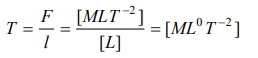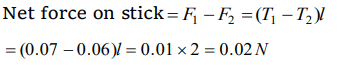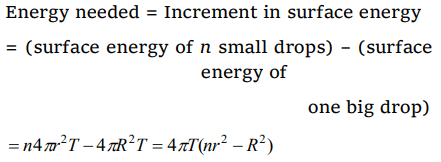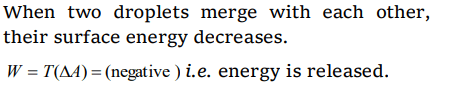## Mechanical Properties of Fluids Questions and Answers Part-5

1. The property utilized in the manufacture of lead shots is
a) Specific weight of liquid lead
b) Specific gravity of liquid lead
d) Surface tension of liquid lead

Explanation: Surface tension of liquid lead

2.The dimensions of surface tension are
a) $\left[MLT^{-1}\right]$
b) $\left[ML^{2}T^{-2}\right]$
c) $\left[ML^{0}T^{-2}\right]$
d) $\left[ML^{-1}T^{-2}\right]$

Explanation:3. A wooden stick 2m long is floating on the surface of water. The surface tension of water 0.07 N/m. By putting soap solution on one side of the sticks the surface tension is reduced to 0.06 N/m. The net force on the stick will be
a) 0.07 N
b) 0.06 N
c) 0.01 N
d) 0.02 N

Explanation:4. The force required to take away a flat circular plate of radius 2 cm from the surface of water, will be (the surface tension of water is 70 dyne/cm)
a) $280\pi$  dyne
b) $250\pi$  dyne
c) $140\pi$  dyne
d) $210\pi$  dyne

Explanation: Force required, F = 2 $\pi$ rT = 2 $\pi$ * 2 * 70 = 280 $\pi$ Dyne

5.Surface tension may be defined as
a) The work done per unit area in increasing the surface area of a liquid under isothermal condition
b) The work done per unit area in increasing the surface area of a liquid under adiabatic condition
c) The work done per unit area in increasing the surface area of a liquid under both isothermal and adiabatic conditions
d) Free surface energy per unit volume

Explanation: The work done per unit area in increasing the surface area of a liquid under isothermal condition

6.Energy needed in breaking a drop of radius R into n drops of radii r is given by
a) $4\pi T\left(nr^{2}-R^{2}\right)$
b) $\frac{4}{3}\pi \left(r^{3}n-R^{2}\right)$
c) $4\pi T\left(R^{2}-nr^{2}\right)$
d) $4\pi T\left(nr^{2}+R^{2}\right)$

Explanation:7.The potential energy of a molecule on the surface of liquid compared to one inside the liquid is
a) Zero
b) Smaller
c) The same
d) Greater

Explanation: Greater

8. Two droplets merge with each other and forms a large droplet. In this process
a) Energy is liberated
b) Energy is absorbed
c) Neither liberated nor absorbed
d) Some mass is converted into energy

Explanation:9. A drop of liquid of diameter 2.8 mm breaks up into 125 identical drops. The change in energy is nearly (S.T. of liquid =75 dynes/cm)
a) Zero
b) 19 erg
c) 46 erg
d) 74 erga) $4\pi r^{2} T$
b) $2\pi r^{2} T$
c) $12\pi r^{2} T$
d) $24\pi r^{2} T$